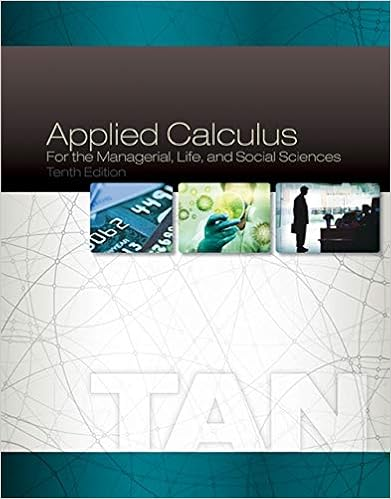# 2 calculate the total direct manufacturing labor

• Homework Help
• 72
• 96% (238) 229 out of 238 people found this document helpful

This preview shows page 55 - 59 out of 72 pages.

##### We have textbook solutions for you!
The document you are viewing contains questions related to this textbook.The document you are viewing contains questions related to this textbook.
Chapter 2 / Exercise 61
Applied Calculus for the Managerial, Life, and Social Sciences
TanExpert Verified
2.Calculate the total direct manufacturing labor price and efficiency variances.3.For the 50 cakes, what is the total actual amount of direct manufacturing labor used? What isthe actual direct manufacturing labor input mix percentage? What is the budgeted amount ofGabrielle’s and Joseph’s labor that should have been used for the 50 cakes?4.Calculate the total direct manufacturing labor mix and yield variances. How do these num-bers relate to the total direct manufacturing labor efficiency variance? What do these vari-ances tell you?SOLUTION
##### We have textbook solutions for you!
The document you are viewing contains questions related to this textbook.The document you are viewing contains questions related to this textbook.
Chapter 2 / Exercise 61
Applied Calculus for the Managerial, Life, and Social Sciences
TanExpert Verified
(35 min.) Direct manufacturing labor variances: price, efficiency, mix and yield1.Gabrielle (\$25 × 3 hrs.)\$ 75Joseph (\$15 × 3 hrs.)45Cost per cake\$ 120Number of cakes× 50 unitsTotal budgeted cost\$ 6,0002.   Solution Exhibit 7-44A presents the total price variance (\$0), the total efficiency variance(\$25 F), and the total flexible-budget variance (\$25 F). Total direct labor price variance can also be computed as:= ActualBudgetedprice of inputprice of input× Actual quantityof inputGabrielle= (\$25 – \$25) × 140 = \$0Joseph= (\$15 – \$15) × 165 = 0Total direct labor price variance \$0Total direct labor efficiency variance can also be computed as:= Actual quantityBudgeted quantity of inputof inputallowed for actual output× Budgetedprice of inputGabrielle = (140 150) × \$25.00 = \$250 FJoseph= (165 150) × \$15.00 = 225UTotal direct labor efficiency variance \$ 25FDirect laborpricevariance forDirect laborefficiencyvariance for
SOLUTION EXHIBIT 7-44AColumnar Presentation of Direct Labor Price and Efficiency Variances for Elena Martinez Wed-ding CakesActual CostsIncurred(Actual Input Quantity× Actual Price)(1)Actual Input Quantity× Budgeted Price(2)Flexible Budget(Budgeted Input QuantityAllowed for Actual Output× Budgeted Price)(3)GabrielleJoseph140 × \$25 = \$3,500165 × \$15 = 2,475\$5,975140 × \$25 = \$3,500165 × \$15 = 2,475\$5,975150 × \$25 = \$3,750150 × \$15 = 2,250\$6,000\$0\$25 FTotal price variance       \$25 FTotal flexible-budget varianceF = favorable effect on operating income; U = unfavorable effect on operating income3.Actual Quan-tityof InputActualMixBudgeted Quantity of Input for Actual OutputBudgetedMixGabrielle140 hours45.9%3 hours × 50 units = 150 hours50%Joseph165 hours54.1%3 hours × 50 units = 150hours50%Total305hours100.0%300hours 100%4. Solution Exhibit 7-44B presents the total direct labor yield and mix variances for Elena Mar-tinez Wedding Cakes. The total direct labor yield variance can also be computed as the sum of the direct laboryield variances for each input:=×× Gabrielle = (305 – 300) × 0.50 × \$25 = 5 × 0.50 × \$25 = \$ 62.50 U Joseph = (305 – 300) × 0.50 × \$15 = 5 × 0.50 × \$15 = 37.50 UTotal direct labor yield variance \$ 100.00UThe total direct labor mix variance can also be computed as the sum of the direct labor mix vari-ances for each input:DirectlaboryieldActualtotalquantityof allBudgeted totalquantity of alldirect labor inputsBudgeteddirectlabor inputBudgetedprice ofdirectDirectlabormix
=×× Gabrielle = (0.459 – 0.50) × 305 × \$25 = – 0.041 × 305 × \$25 = \$312.63 F                       Joseph = (0.541 – 0.50) × 305 × \$15 = 0.041 × 305 × \$15 = 187.58UTotal direct labor mix variance \$125.05Fround to \$125 F
•••